Balbharti Maharashtra Board Class 5 Maths Solutions Chapter 7 Circles Problem Set 31 Textbook Exercise Important Questions and Answers.

## Maharashtra State Board Class 5 Maths Solutions Chapter 7 Circles Problem Set 31

Question 1.
In the figure given alongside, points S, L, M, and N are on the circle. Answer the questions with the help of the diagram.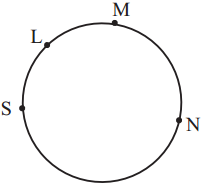(1) Write the names of the arcs with end-points S and M.
Arcs with the end-points S and M are, arc SLM and arc SNM.

(2) Write the names of the arcs with the end-points L and N.
Arcs with the end-points L and N are, arc LMN and arc LSN.

Question 2.
Write the names of arcs that points A, B, C, and D in the given circle give rise to.
Arcs with end-points A and C are, arc ABC and arc ADC.
Arcs with end-points B and D are, arc BAD and arc BCD.

Question 3.
Give the names of the arcs that are made by points P, Q, R, S, and T in the figure.
Taking end-points : P and R, arc PQR, arc PTR.
Taking end-points: Q and S, arc QRS, arc QTS
Taking end-points : R and T, arc RST, arc RPT
Taking end-points : S and P, arc STP, arc SRP
Taking end-points: Q and T, arc QPT, arc QSTQuestion 4.
Measure and note down the circumference of different circular objects. (It is convenient to use a measuring tape for this purpose.)

Question 1.
Draw circles with the radii given below:
(1) 1.2 cm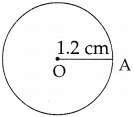(2) 2.5 cm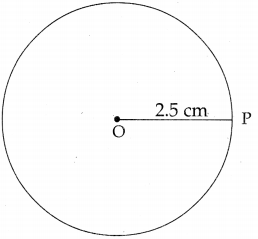(3) 3.3 cm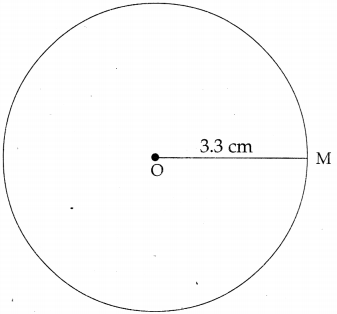Question 2.
Write true or false for the following statements:
(1) Longest chord is a diameter.
(2) Centre is not lying on the diameter.
(3) All chords are of equal length.
(4) All chords passes through the centre.
(1) True
(2) False
(3) False
(4) FalseQuestion 3.
Match the cplumns (A) and (B):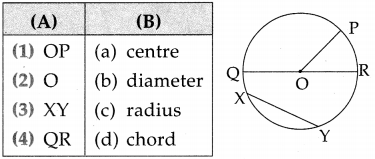(1) ↔ (c),
(2) ↔ (a),
(3) ↔ (d),
(4) ↔ (b)

Question 4.
Complete the following table by filling in the blanks: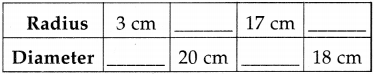(1) 6 cm
(2) 10 cm
(3) 34 cm
(4) 9 cmQuestion 5.
From the following figure, fill in the blanks: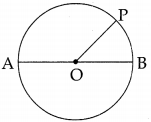(1) If OP = 4cm then AB = _________ cm, OA = _________ cm, OB = _________ cm.
(2) If AB = 10 cm then OA = _________ cm, OB = _________ cm, OP = _________ cm.
(1) AB = 8 cm, OA = 4 cm, OB = 4 cm
(2) OA = 5 cm, OB = 5 cm, OP = 5 cm

Question 6.
In the table below, write the names of the points in the interior and exterior of the circle and those on the circle.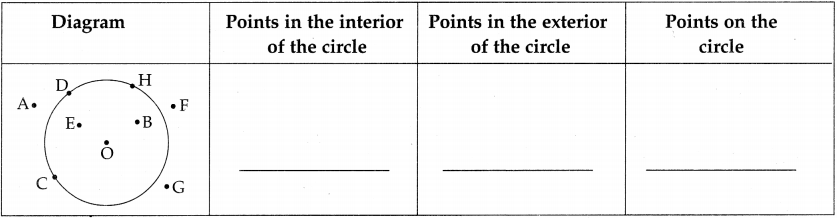Points in the exterior of the circle are A, F and G.
Points in the interior of the circle are O, E and B, and Points on the circle are C, D and H.Question 7.
Radius of a circle with centre P is 4 cm. Fill in the blanks.
(1) The, point A is at a distance of 5 cm from the centre P. Flence the point A lies in the ________ of the circle.
(2) Point B is at a distance of 4 cm. from the centre P. Hence the point B lies ________ circle.
(3) Point C lies at a distance 3 cm from the centre P. Hence it lies in the ________ of the circle.
(1) Exterior
(2) on the circle
(3) interior

Question 8.
Solve the following:
(1) What is the length of the diameter of a circle of radius 6 cm?
(2) What is the length of the radius of a circle of diameter 14 cm?
(3) Give the names of the arcs that are made by points X, Y, Z and W in this picture.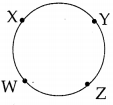(4) Give the names of the arc that are made by points E, F, G and H, taking end points E and G.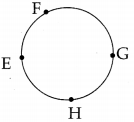(5) If the diameter of a circle is 7 cm. what is the length of the circumference? (Use measure tap)